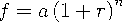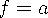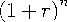Functions and CALL Routines

# COMPOUND Function

Returns compound interest parameters.
 Category: Financial

## Syntax

 COMPOUND(a,f,r,n)

### Arguments

a

is numeric, and specifies the initial amount.

 Range: a0
f

is numeric, and specifies the future amount (at the end of n periods).

 Range: f0
r

is numeric, and specifies the periodic interest rate expressed as a fraction.

 Range: r0
n

is an integer, and specifies the number of compounding periods.

 Range: n0

The COMPOUND function returns the missing argument in the list of four arguments from a compound interest calculation. The arguments are related by the following equation:One missing argument must be provided. A compound interest parameter is then calculated from the remaining three values. No adjustment is made to convert the results to round numbers.

If n=0, thenandare equal to 1.

Note:   If you choose r as your missing value, then COMPOUND returns an error.The accumulated value of an investment of \$2000 at a nominal annual interest rate of 9 percent, compounded monthly after 30 months, can be expressed as

`   future=compound(2000,.,0.09/12,30);`

The value returned is 2502.54. The second argument has been set to missing, indicating that the future amount is to be calculated. The 9 percent nominal annual rate has been converted to a monthly rate of 0.09/12. The rate argument is the fractional (not the percentage) interest rate per compounding period.Previous Page | Next Page | Top of Page